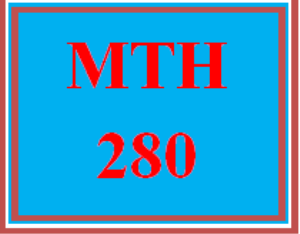# MTH 280 Week 5 MyMathLab Study Plan for Weekly Checkpoint

PLDZ-9132 Free In Stock
\$ 0.00 USD
Description

***************************************************************

~~~~~~~~~~~~~~~~~~~~~~~~~~~~~~~~~~~~~~~~~~~~~~~~~

You can buy more tutorials at a more favorable price from the below link.

***************************************************************

---------------------------------------------------------------------------------------------------------------------

MTH 280 Week  5 MyMathLab Study Plan for Weekly Checkpoint

4.1 Extreme Values of Functions

Find extreme values and where they occur.

Find absolute extrema on finite closed intervals.

Find all critical points for a function.

Find critical points and local extrema.

Solve applications involving extreme values of functions.

4.2 The Mean Value Theorem

Find the values that satisfy the conclusion of the mean value theorem.

Find the values of functions given the derivatives.

Find functions from given derivatives at a given point.

Solve applications involving the mean value theorem.

4.3 Monotonic Functions and the First Derivative Test

Given the derivative, find increasing and decreasing intervals and extrema.

Given the function, find increasing and decreasing intervals and extrema.

Find local and absolute extrema in a given domain.

4.4 Concavity and Curve Sketching

Identify the inflection points, local extrema, and concavity from graphs.

Graph functions, and find any local extrema and inflection points.

Sketch the general graph of functions given the equation of the derivatives.

Graph f(x) given the graphs of f'(x) and f”(x).

Graph rational functions.

Graph functions given certain conditions.

Solve applications involving concavity and curve sketching.

Recent Reviews Write a Review
0 0 0 0 reviews